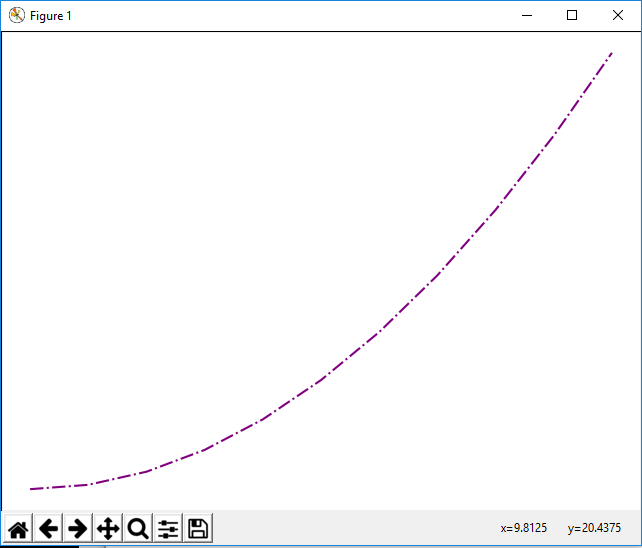﻿ How to Change the Line Width of a Graph Plot in Matplotlib with Python ﻿# How to Change the Line Width of a Graph Plot in Matplotlib with PythonIn this article, we show how to change the line style of a graph plot in matplotlib with Python.

By line style, what is meant is whether the line is solid, dotted, dashed, or with a dot-dash style.

By default, lines are solid in matplotlib. So if you want a graph plot to be solid, you do not have to specify the line style, as it is solid by default.

However, if you want the line to be anything else, such as dotted, dashed, etc, then you must know how to change the line style in matplotlib.

So we are able to change the style of a line with the linestyle attribute. We can set this equal to various elements.

In the following code below, we set the style of the line to be be dotted.

So the first thing we have to do is import matplotlib. We do this with the line, import matplotlib.pyplot as plt

We then create a variable fig, and set it equal to, plt.figure()

This creates a figure object, which of course is initially empty, because we haven't populated it with anything. We then add axes to this figure. We then have our x coordinates that range from 0 to 10.

We then plot the square of the x coordinates.

It is with the plot() function that we specify the line style of the plot. We do this with the linestyle attribute. In this case, we set the line style equal to dotted, making the graph plot a dotted line.

Lastly, we show the figure with the show() function.

This works if you're using a python IDE other than jupyter notebooks. If you are using jupyter notebooks, then you would not use, plt.show(). Instead you would specify in the code right after importing matplotlib, %matplotlib inline

This line allows the figure of a graph to be shown with jupyter notebooks.

After running the following code above, we get the following figure with a dotted line shown in the image below.There are several other options you can choose, including, dashed and dashdot, lines.

If we change the linestyle to dashed, we get the following figure shown below.IF we change the linestyle to dashdot, we get the following figure shown below.And this is how to chnage the line style of a graph plot in matplotlib with Python.

Related Resources

How to Randomly Select From or Shuffle a List in Python